# Methods and formulas for coefficients in Fit Mixed Effects Model

Select the method or formula of your choice.

## Coefficients in mixed models

In matrix terms, the vector of estimated coefficients follows:

For further details on the notation, go to the Methods section.

## Standard errors of the coefficients in mixed models

The standard errors of the coefficients depend on the test method for the fixed effects. For further details on the notation, go to the Methods section and the Tests of Fixed Effects section.

### Kenward-Roger's approximation

The standard errors of the estimated coefficients are the square roots of the dialgonal elements of the matrix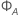.

where

### Satterthwaite Approximation

The standard errors are the square roots of the diagonal elements of the matrix,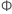.

where

## Degrees of freedom for coefficients

The following hypotheses are for the tests of the coefficients:
The following equations give the degrees of freedom for thecoefficient: where

For further details on the notation, go to the Methods section and the Tests of Fixed Effects section.

## Confidence intervals for coefficients

The (1 − α)% confidence limits for thecoefficient has the following equation:

### Notation

TermDescription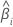the estimated coefficient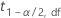the 1 − α/2 percentile from the t distribution with df degrees of freedom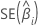the standard error of the estimated coefficient

## T-value

### Notation

TermDescription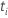test statistic for thecoefficientestimated coefficientstandard error of theestimated coefficient

## p-value (P)

The following equation gives the two-sided p-value for the null hypothesis that a coefficient equals 0:

### Notation

TermDescription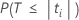The probability that under the null hypothesis T is less than the absolute value of the calculated. Here, T follows a t-distribution with df degrees of freedom.The t value for thecoefficient.